Chapter 9 Electromagnetic Waves

# 9.1 Review of wavesFigure 1. There are at least four types of waves in this picture—only the water waves are evident. There are also sound waves, light waves, and waves on the guitar strings. (credit: John Norton)

What do an ocean buoy, a child in a swing, the cone inside a speaker, a guitar, atoms in a crystal, the motion of chest cavities, and the beating of hearts all have in common? They all oscillate—-that is, they move back and forth between two points. Many systems oscillate, and they have certain characteristics in common. All oscillations involve force and energy. You push a child in a swing to get the motion started. The energy of atoms vibrating in a crystal can be increased with heat. You put energy into a guitar string when you pluck it.

Some oscillations create waves. A guitar creates sound waves. You can make water waves in a swimming pool by slapping the water with your hand. You can no doubt think of other types of waves. Some, such as water waves, are visible. Some, such as sound waves, are not. But every wave is a disturbance that moves from its source and carries energy. Other examples of waves include earthquakes and visible light. Even subatomic particles, such as electrons, can behave like waves.

By studying oscillatory motion and waves, we shall find that a small number of underlying principles describe all of them and that wave phenomena are more common than you have ever imagined. We begin by studying the type of force that underlies the simplest oscillations and waves. We will then expand our exploration of oscillatory motion and waves to include concepts such as simple harmonic motion, uniform circular motion, and damped harmonic motion. Finally, we will explore what happens when two or more waves share the same space, in the phenomena known as superposition and interference.

These next few sections are from chapter 16 in the original Open Stax College Physics.

## Glossary

oscillate
moving back and forth regularly between two points
wave
a disturbance that moves from its source and carries energy

### Summary

• Observe the vibrations of a guitar string.
• Determine the frequency of oscillations.

When you pluck a guitar string, the resulting sound has a steady tone and lasts a long time. Each successive vibration of the string takes the same time as the previous one. We define periodic motion to be a motion that repeats itself at regular time intervals, such as exhibited by the guitar string or by an object on a spring moving up and down. The time to complete one oscillation remains constant and is called the period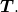Its units are usually seconds, but may be any convenient unit of time. The word period refers to the time for some event whether repetitive or not; but we shall be primarily interested in periodic motion, which is by definition repetitive. A concept closely related to period is the frequency of an event. For example, if you get a pay check twice a month, the frequency of payment is two per month and the period between checks is half a month. Frequency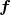is defined to be the number of events per unit time. For periodic motion, frequency is the number of oscillations per unit time. The relationship between frequency and period is

T = 1/ f     or     f = 1 / T

The SI unit for frequency is the cycle per second, which is defined to be a hertz (Hz):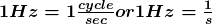A cycle is one complete oscillation. Note that a vibration can be a single or multiple event, whereas oscillations are usually repetitive for a significant number of cycles.

### Example 1: Determine the Frequency of Two Oscillations: Medical Ultrasound and the Period Middle C

We can use the formulas presented in this module to determine both the frequency based on known oscillations and the oscillation based on a known frequency. Let’s try one example of each. (a) A medical imaging device produces ultrasound by oscillating with a period of 0.400 µs. What is the frequency of this oscillation? (b) The frequency of middle C on a typical musical instrument is 264 Hz. What is the time for one complete oscillation?

Strategy

Both questions (a) and (b) can be answered using the relationship between period and frequency. In question (a), the period is given and we are asked to find frequency f. In question (b), the frequency is given and we are asked to find the period T.

Solution a

1. Substitute 0.400 μs  for T  in f = 1/T.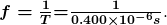Solve to find

f = 2.50 x 106 Hz.

Discussion a

The frequency of sound found in (a) is much higher than the highest frequency that humans can hear and, therefore, is called ultrasound. Appropriate oscillations at this frequency generate ultrasound used for noninvasive medical diagnoses, such as observations of a fetus in the womb.

Solution b

1. Identify the known values:

The time for one complete oscillation is the period T where f = 1/T.

2. Solve for T.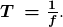3. Substitute the given value for the frequency into the resulting expression: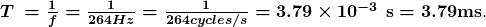Discussion

The period found in (b) is the time per cycle, but this value is often quoted as simply the time in convenient units (ms or milliseconds in this case).

1: Identify an event in your life (such as receiving a paycheck) that occurs regularly. Identify both the period and frequency of this event.

# Section Summary

• Periodic motion is a repetitious oscillation.
• The time for one oscillation is the period T.
• The number of oscillations per unit time is the frequency f.
• These quantities are related by T = 1/ f   or  f = 1 / T

### Problems & Exercises

1: What is the period of 60 Hz  (sixty hertz) electrical power?

2: If your heart rate is 150 beats per minute during strenuous exercise, what is the time per beat in  seconds?

3: Find the frequency of a tuning fork that takes 2.50 x 10-3 s to complete one oscillation.

4: A stroboscope is set to flash every 8.00 x 10-5 s.  What is the frequency of the flashes?

6: Engineering Application

Each piston of an engine makes a sharp sound every other revolution of the engine. (a) How fast is a race car going if its eight-cylinder engine emits a sound of frequency 750 Hz, given that the engine makes 2000 revolutions per kilometer? (b) At how many revolutions per minute is the engine rotating?

## Glossary

period
time it takes to complete one oscillation
periodic motion
motion that repeats itself at regular time intervals
frequency
number of events per unit of time

### Solutions

1: I visit my parents for dinner every other Sunday. The frequency of my visits is 26 per calendar year. The period is two weeks.

Problems & Exercises

1: 16.7 ms

2:  1 minute = 60 seconds     60 seconds / 150  beats = 0.4 seconds for one beat

3: 400 Hz

4: 12,500 Hz

6: (a) 93.8 m/s  (b) 11.3 x 103 rev/min or rpm.